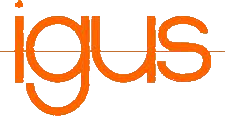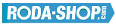# Remote ControlManufacturer Product Image Item Name Price### 61702

Thin Section Bearings Chrome Steel Open(No Shields) Dimension d=15.00 Dimension D=21.00 Dimension B=4.00 Dinamic Load Rating=937 Static Load Rating=582 Weight=0.004gr. Speed limit Grease=11.0 Speed limit Oil=13.0
inside diameter = 15.00
outside diameter = 21.00
width = 4.00
€4.54### 61703-2RS

Thin Section Bearings Chrome Steel Rubber Seals on Both sides Dimension d=17.00 Dimension D=23.00 Dimension B=4.00 Dinamic Load Rating=1000 Static Load Rating=658 Weight=0.005gr. Speed limit Grease=9.5 Speed limit Oil=11.0
inside diameter = 17.00
outside diameter = 23.00
width = 4.00
€4.54### 61703-2Z

Thin Section Bearings Chrome Steel Metallic Shields on both sides Dimension d=17.00 Dimension D=23.00 Dimension B=4.00 Dinamic Load Rating=1000 Static Load Rating=658 Weight=0.005gr. Speed limit Grease=9.5 Speed limit Oil=11.0
inside diameter = 17.00
outside diameter = 23.00
width = 4.00
€4.54### 61800

Thin Section Bearings Chrome Steel Open(No Shields) Dimension d=10.00 Dimension D=19.00 Dimension B=5.00 Dinamic Load Rating=1716 Static Load Rating=840 Weight=0.006gr. Speed limit Grease=37.0 Speed limit Oil=43.0
inside diameter = 10.00
outside diameter = 19.00
width = 5.00
€4.24### 61800-2RS

Thin Section Bearings Chrome Steel Rubber Seals on Both sides Dimension d=10.00 Dimension D=19.00 Dimension B=5.00 Dinamic Load Rating=1716 Static Load Rating=840 Weight=0.006gr. Speed limit Grease=37.0 Speed limit Oil=43.0
inside diameter = 10.00
outside diameter = 19.00
width = 5.00
€4.24### 61800-2Z

Thin Section Bearings Chrome Steel Metallic Shields on both sides Dimension d=10.00 Dimension D=19.00 Dimension B=5.00 Dinamic Load Rating=1716 Static Load Rating=840 Weight=0.006gr. Speed limit Grease=37.0 Speed limit Oil=43.0
inside diameter = 10.00
outside diameter = 19.00
width = 5.00
€4.24### 61801-2RS

Thin Section Bearings Chrome Steel Rubber Seals on Both sides Dimension d=12.00 Dimension D=21.00 Dimension B=5.00 Dinamic Load Rating=1915 Static Load Rating=1041 Weight=0.007gr. Speed limit Grease=33.0 Speed limit Oil=39.0
inside diameter = 12.00
outside diameter = 21.00
width = 5.00
€4.24### 61801-2Z

Thin Section Bearings Chrome Steel Metallic Shields on both sides Dimension d=12.00 Dimension D=21.00 Dimension B=5.00 Dinamic Load Rating=1915 Static Load Rating=1041 Weight=0.007gr. Speed limit Grease=33.0 Speed limit Oil=39.0
inside diameter = 12.00
outside diameter = 21.00
width = 5.00
€4.24### 61802-2RS

Thin Section Bearings Chrome Steel Rubber Seals on Both sides Dimension d=15.00 Dimension D=24.00 Dimension B=5.00 Dinamic Load Rating=2073 Static Load Rating=1253 Weight=0.009gr. Speed limit Grease=28.0 Speed limit Oil=33.0
inside diameter = 15.00
outside diameter = 24.00
width = 5.00
€4.54### 61802-2Z

Thin Section Bearings Chrome Steel Metallic Shields on both sides Dimension d=15.00 Dimension D=24.00 Dimension B=5.00 Dinamic Load Rating=2073 Static Load Rating=1253 Weight=0.009gr. Speed limit Grease=28.0 Speed limit Oil=33.0
inside diameter = 15.00
outside diameter = 24.00
width = 5.00
€4.54